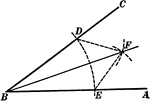### Construction Of Angle Bisector

Illustration showing how to construct the bisector of an angle.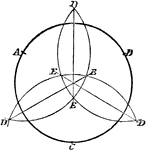### Construction of Circle Given 3 Points

Illustration used to construct a circle when given three points.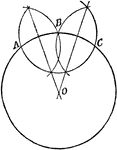### Center of a Circle

Illustration used to find the center of a circle.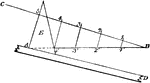### Construction Used to Divide a Line Into Equal Parts

Illustration used to show how to divide a line into any number of equal parts by construction.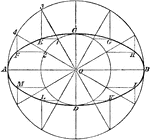### Construction of Ellipse by Describing Circles

Illustration used to draw a an ellipse using string and pins by describing a circles with diameters…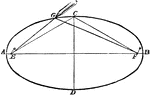### Construction of Ellipse Using String

Illustration used to draw a an ellipse using string and pins.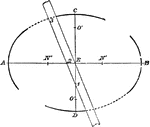### Construction of Ellipse

Illustration used to draw a an ellipse with major axis AB and minor axis CD.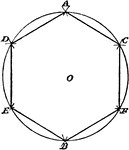### Construction of Regular Hexagon

Illustration used to construct a regular hexagon on a given line.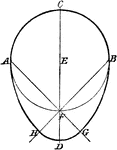### Construction of Oval

Illustration used to construct and oval when given the width.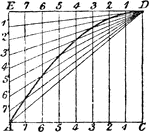### Construction of Parabola Given Base and Height

Illustration used to draw a parabola when given the base and height.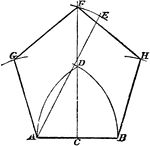### Construction of Regular Pentagon

Illustration used to construct a regular pentagon on a given line.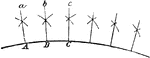### Construction of Radii of a Circle

Illustration used to draw lines which are radii of a circle where the center is inaccessible.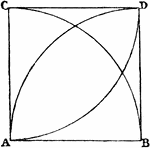### Construction of Square on Given Line

Illustration used to construct a square on a given line.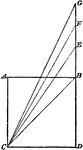### Construction of Multiple of a Square

Illustration used to construct a square that shall be a multiple of any given square.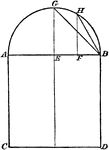### Construction of Proportional Square

Illustration used to construct a square that shall be in proportion to a given square.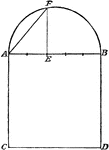### Construction of Proportional Square

Illustration used to construct a square that shall be in proportion to a given square.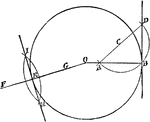### Construction of Tangent to a Circle

Illustration used to draw a tangent to a circle.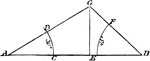### Construction of Triangle Given Angles and Base

Illustration used to construct a triangle given the length of the base and the two base angles.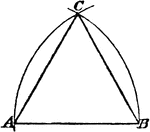### Construction of an Equilateral Triangle

Illustration used to construct an equilateral triangle on a given base.